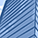## Solve for confidence interval half-width in in PROC POWER ONESAMPLEFREQ

It would be wonderful if you could have SAS tell you the half width of a confidence interval for a proportion if given a coverage probability, true outcome proportion, and sample size in PROC POWER ONESAMPLEFREQ. This would be useful for choosing a sample size for a single-arm clinical trial.

Example code:

``````proc power;
onesamplefreq ci=wilson /* or exact, wald, etc. */
halfwidth = . /* want to solve for this! */
proportion = .5 .7 .85
ntotal = 25 50 75
probwidth = .95;
run;``````

1 CommentSAS Super FREQ

For the confidence interval precision analysis in PROC POWER, the only thing you can solve is PROBWIDTH. You can provide a list of values for HALFWIDTH and ask the procedure to solve for PROBWIDTH, the one that results in a value that is closest to the desired PROBWIDTH value would be the HALDWIDTH value you are looking for. It might take several trial and error to get what you want.

Jill Tao

SAS Technical Support